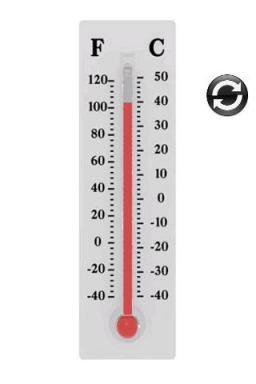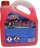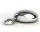# Degrees 2

The temperature was 3°F and fell four degrees Fahrenheit. What is actually temperature?

t =  -1 °F

### Step-by-step explanation:

$t=3-4=-1\text{°F}$Did you find an error or inaccuracy? Feel free to write us. Thank you!## Related math problems and questions:

• The outdoorThe outdoor temperature was 60 degrees Fahrenheit at midnight. The temperature went down 3 degrees each hour for the next 4 hours. What was the temperature at 4 A. M. ?
• Temperature rise and fallOn Friday, the temperature was 82°F. The temperature changed by –2°F on Saturday, and then it changed by 5°F on Sunday. What was the temperature on Sunday? How did the temperature change? Note we consider the mathematical problem formulated in this way to
• The temperature 3The temperature is -12 degrees Fahrenheit. During the following 5 hours, the temperature decrease by 23 degrees Fahrenheit. What is the weather at 11 pm?
• Temperature change 3At 2 PM, The temperature was 76 degrees Fahrenheit. At 8 PM, the temperature was 58 degrees Fahrenheit. What was the change in temperature?
• NoontimeIn the morning temperature was 110°F. By noontime, it has gone up by 15°F. What was the noon temperature? (Give your answer in °F)
• Temperature 2Sunday's high temperature was 3 degrees higher than Saturday's. On Monday, the temperature fell 5 degrees, then rose 7 degrees on Tuesday and 4 more on Wednesday. Then it fell 17 degrees to a record low of 31 on Thursday. What was the temperature on Satur
• Temperature changeThe mean temperature change is -3.2°F per day for five days. What is the total change over the five day period?
• Outdoor temperatureThe outdoor temperature was 8 degrees at midnight. The temperature declined 5 degrees during each of the next 3 hours. What was the temperature at 3am?
• The temperature 10The temperature in a freezer is -15°C, and it increases by 3°C.
• Mixing Celsius and FahrenheitAdd up three temperatures: 5°F +6°F +0°C
• The temperature 2The temperature of Siberia fell13 degrees below 2/3 degrees centigrad. What is the temperature there now?
• ThermometerThe thermometer showed -12 degrees Celsius in the morning then the temperature rises by 4 degrees and later again increased by 2 degrees at the evening has fallen by 5 degrees and then falls 3 degrees. What end temperature does the thermometer show?
• Change in temperatureStarting temperature is 21°C, the highest temperature is 32°C. What is the change in temperature?
• Celsius degreesThe temperature on Monday was 5 celsius. The temperature on Thursday was 7 degrees less than the temperature on Monday. What was the temperature on Thursday?
• OpheliaOphelia recorded the temperature of a cold store every two hours. 1. At 6 am, it was -4°C, and at 8 am, it was -1°C. By how much did the temperature rise? 2. The temperature went up by 5°C in the next two hours. .What was the temperature at 10 am?
• The temperature 6The temperature was 47°F on Thursday and 60°F on Friday. How much did the temperature rise?
• Melting pointThe melting point of mercury is -36°F and its boiling point is 672°F. What is the difference between the boiling point and the melting point?